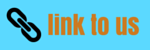# Factorization Test Paper

In this page we have Factorization Test Paper . Hope you like them and do not forget to like , social share and comment at the end of the page.
Question 1
The sum of (x + 5) observations is x4 – 625. Find the mean of the observations.
Question 2
The height of a triangle is x4 + y4 and its base is 14xy. Find the area of the triangle.
Question 3
Factorise
p4 – (p – q)4

Question 4
The area of a circle is given by the expression πx2 + 6πx + 9π. Find the radius of the circle.
Question 5
Factorize the expressions.
63p2q2 r2 s – 9pq2 r2 s2 + 15p2qr2 s2 – 60p2 q2 rs2